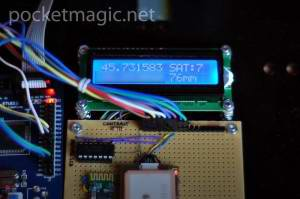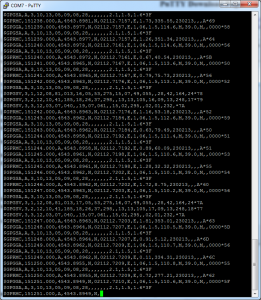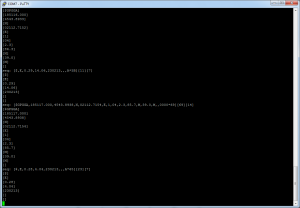# NMEA GPS Library for AVR Microcontrollers

Recently I have developed a GPS NMEA Parser for an embedded system using the ATMega128 microcontroller, and decided to pack everything together as a GPS open source library for AVR Microcontrollers. The code is highly optimized to load the microprocessor as low as possible (both in terms of storage and CPU load), so the serial data is parsed as it gets in, without using redundant data structures or operations.### Short Intro. NMEA Sentences

Most GPS Modules output serial data, using the RX TX pins, and the UART protocol. The data itself is in the NMEA format, a CSV (comma separated value) format with predefined keywords. One such set of NMEA CSV values, that starts with \$ and ends with EOL, is called a [b]NMEA sentence[/b].
The NMEA sentence is a comma separated list of values which contains information on a single GPS reading. There are a number of sentence types, each with its own, variable number of values, as defined by the NMEA protocol, such as the GPRMC (Recommended minimum specific GPS/Transit data) sentence, the GPGGA (Global Positioning System Fix Data) or the GPGSV (GPS Satellites in view) just to name a few. See this page for more details on the NMEA sentences.

### More details

The library consists of two files, nmea.cpp and nmea.h defining a C++ class, named NMEA. The GPS data is provided to a instance of this class (an object) using the feed function int fusedata(char c); . As you can see this function takes one char, and must be called each time serial data is received from the GPS module.
For AVR – GPS communication, I wrote another handy piece of code, an UART library allowing both polling based and interrupt based information exchange. As this is written as a C++ class (uart.cpp and uart.h), you’ll be able to instantiate any number of objects, providing only the UART ports you are planning to use. For the Atmega128 this is extremely useful as this chip supports two UART gateways: uart0 (rx0/tx0) on pins PE0+PE1 and uart1 (rx1/tx1) on PD2+PD3. I hooked the GPS up to uart0, and for uart1 I connected an UART Bluetooth module. This proved extremely useful for debugging my code, while implementing this NMEA parser library, as there were many situations that required careful analysis of the algorithm and its results (string parsing, string cutting, etc). Connecting the Bluetooth module to the PC, a simple terminal application such as Putty can be used to visualize strings received from the microcontroller board:With only two objects, we can handle both the GPS and the Bluetooth modules easy:

```UART	uart0, // gps module
uart1; // bluetooth module
//USART0 RX interrupt this code is executed when we recieve a character
ISR(USART0_RX_vect){
volatile char c = UDR0; //Read the value out of the UART buffer
nmea.fusedata(c);
}

//USART1 RX interrupt this code is executed when we recieve a character
ISR(USART1_RX_vect){
volatile char c = UDR1; //Read the value out of the UART buffer
if (c == '1') _delay_ms(10000); // pause execution when we send char '1' for debugging
}
uart0.Init(0,4800, true); //uart0: GPS
uart1.Init(1,9600, true); //uart1: Bluetooth
```

The serial data is assembled on the fly, without using any redundant buffers. When a sentence is complete (one that starts with \$, ending in EOL), all processing is done on this temporary buffer that we’ve built: checksum computation, extracting sentence “words” (the CSV values), and so on.
When a new sentence is fully assembled using the fusedata function, the code calls parsedata. This function in turn, splits the sentences and interprets the data. Here is part of the parser function, handling both the \$GPRMC NMEA sentence:

```/* \$GPGGA
* \$GPGGA,hhmmss.ss,llll.ll,a,yyyyy.yy,a,x,xx,x.x,x.x,M,x.x,M,x.x,xxxx*hh
* ex: \$GPGGA,230600.501,4543.8895,N,02112.7238,E,1,03,3.3,96.7,M,39.0,M,,0000*6A,
*
* WORDS:
*  1    = UTC of Position
*  2    = Latitude
*  3    = N or S
*  4    = Longitude
*  5    = E or W
*  6    = GPS quality indicator (0=invalid; 1=GPS fix; 2=Diff. GPS fix)
*  7    = Number of satellites in use [not those in view]
*  8    = Horizontal dilution of position
*  9    = Antenna altitude above/below mean sea level (geoid)
*  10   = Meters  (Antenna height unit)
*  11   = Geoidal separation (Diff. between WGS-84 earth ellipsoid and mean sea level.
*      -geoid is below WGS-84 ellipsoid)
*  12   = Meters  (Units of geoidal separation)
*  13   = Age in seconds since last update from diff. reference station
*  14   = Diff. reference station ID#
*  15   = Checksum
*/
if (mstrcmp(tmp_words, "\$GPGGA") == 0) {
// Check GPS Fix: 0=no fix, 1=GPS fix, 2=Dif. GPS fix
if (tmp_words == '0') {
// clear data
res_fLatitude = 0;
res_fLongitude = 0;
return;
}
// parse time
res_nUTCHour = digit2dec(tmp_words) * 10 + digit2dec(tmp_words);
res_nUTCMin = digit2dec(tmp_words) * 10 + digit2dec(tmp_words);
res_nUTCSec = digit2dec(tmp_words) * 10 + digit2dec(tmp_words);
// parse latitude and longitude in NMEA format
res_fLatitude = string2float(tmp_words);
res_fLongitude = string2float(tmp_words);
// get decimal format
if (tmp_words == 'S') res_fLatitude  *= -1.0;
if (tmp_words == 'W') res_fLongitude *= -1.0;
float degrees = trunc(res_fLatitude / 100.0f);
float minutes = res_fLatitude - (degrees * 100.0f);
res_fLatitude = degrees + minutes / 60.0f;
degrees = trunc(res_fLongitude / 100.0f);
minutes = res_fLongitude - (degrees * 100.0f);
res_fLongitude = degrees + minutes / 60.0f;

// parse number of satellites
res_nSatellitesUsed = (int)string2float(tmp_words);

// parse altitude
res_fAltitude = string2float(tmp_words);

}
```

See the entire code for a better understanding of the logic implied. After parsing, the data is sent to the LCD:

```lcd.lcd_cursor_home();
// use index to change display on every iteration: easy way to show more content on a small screen
if (i%2 == 0) { // show latitude, number of sats, longitude, altitude
lcd.lcd_string_format("%f SATs:%d    \n%f A:%dm    ",
nmea.getLatitude(), nmea.getSatellites(),
nmea.getLongitude(), (int)nmea.getAltitude());
} else { //show speed, bearing, time and date
lcd.lcd_string_format("S:%2.2f B:%2.2f    \n%02d:%02d %02d-%02d-%02d    ",
nmea.getSpeed(), nmea.getBearing(),
nmea.getHour(),nmea.getMinute(),
nmea.getDay(),nmea.getMonth(),nmea.getYear());
}
} else {
lcd.lcd_string("Waiting for GPS  \nfix. No Data.       ");
}
```

The code above uses an index (i) to show more content on the tiny 2×16 LCD. First we show latitude, number of satellites used, longitude and altitude (in meters) and on the next iteration we show speed, bearing UTC time and UTC date. As you can see we call isdataready() function first, to make sure we have a GPS fix and valid data.

### Conversions

To represent the coordinates, NMEA uses DM.MM, (degrees, minutes, decimal minutes) followed by N or S for latitude, and E or W for longitude. Example sentence:
\$GPRMC,230558.501,A,4543.8901,N,02112.7219,E,1.50,181.47,230213,,,A*66,
The GPS Library code converts latitude & longitude obtained from NMEA’s GPGGA or GPRMC to decimal:

```// parse latitude and longitude in NMEA format
res_fLatitude = string2float(tmp_words);
res_fLongitude = string2float(tmp_words);
// get decimal format
if (tmp_words == 'S') res_fLatitude  *= -1.0;
if (tmp_words == 'W') res_fLongitude *= -1.0;
float degrees = trunc(res_fLatitude / 100.0f);
float minutes = res_fLatitude - (degrees * 100.0f);
res_fLatitude = degrees + minutes / 60.0f;
degrees = trunc(res_fLongitude / 100.0f);
minutes = res_fLongitude - (degrees * 100.0f);
res_fLongitude = degrees + minutes / 60.0f;
```

Speed, as returned by GPRMC is in knots/hour. The knot (pronounced not) is a unit of speed equal to one nautical mile (1.852 km) per hour. So to get the speed in km/h we do:

```res_fSpeed = string2float(tmp_words);
res_fSpeed *= 1.852; // convert to km/h
```

The rest is straight forward, but in case you have any questions, feel free o use the comments form below.

Notes:
The LCD is connected using only three wires, using a shift register, as explained here. The pins used are visible in the code:

```lcd.Init(&PORTC,PC0,	// data/rs
&PORTC, PC1,	// clk
&PORTC, PC2);  // lcd e
```

1.Radu Motisan says: